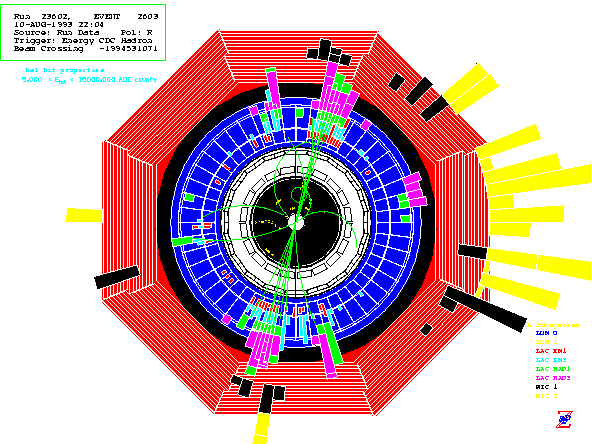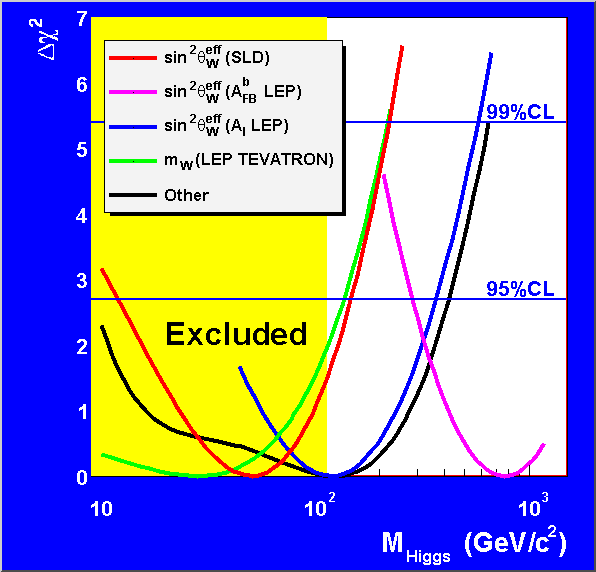Measuring the Strength of the Weak Force Constraining the elusive Higgs particle After 7 years of taking data and 2 more years of analysis, the SLD Experiment at SLAC has finalized its precise measurement of the amount of parity violation when massive Z particles are produced in high energy collisions of electrons and positrons.  This measurement gives the world’s best determination of the weak mixing angle, a fundamental parameter of the theory for electroweak forces that is related to the strength of this force.  SLAC's measurement predicts that the Higgs particle (necessary to explain how particles obtain mass in the Standard Model of particle physics) is light enough to be seen soon at Fermilab’s Tevatron proton collider.  SLAC's measurement is also consistent with recent evidence from CERN's LEP experiments for a light Higgs of mass 115 GeV. The SLD Experiment at SLAC’s Linear Collider (SLC) produced over half a million Z particles in 7 years of operation from 1992-1998.  The SLC was the world’s first and only linear collider, and was a successful prototype for the next generation of electron colliders.  The SLC accelerated and collided beams of electrons and positrons (anti-electrons).  The energies of the 2 colliding beams were tuned to add up to the rest mass of the Z, which is 97 times heavier than the proton, to yield a high Z production rate.SLD Event Display of a Z particle decaying to two quarks.  An electron and positron travelling in opposite directions (perpendicular to this page) collided at the center of the detector and annihilated, creating a Z .  The Z subsequently decayed to a quark and anti-quark, which then hadronized to form two jets of particles traveling in opposite directions.  These are the two jets of green tracks seen in this projection. Electrons, like visible light, can be polarized.  They have an intrinsic spin (angular momentum) which can be oriented parallel (right-handed polarization) or anti-parallel (left-handed polarization) to their momentum direction.  In a similar way that polaroid sunglasses transmit vertically-polarized light preferentially to horizontally-polarized light, the strength of the weak nuclear force (unlike the electromagnetic force or the strong nuclear force) is different for right-handed and left-handed particles.  Neutral Z particles and charged W+ and W-  particles transmit the weak force (radioactivity is an example of a weak force interaction) and do so with different strengths for right-handed and left-handed particles.  The W+ and W- interact only with left-handed particles, while the Z interacts with both but with different strengths.  This left-right asymmetry in weak interactions is known as parity violation.  SLD has measured very precisely the asymmetry in Z interactions with right-handed and left-handed electrons. This parity-violating asymmetry is such that a beam of left-handed electrons will produce 30% more Z particles in collisions with a beam of unpolarized positrons compared to a beam of right-handed electrons.  SLD measures this asymmetry to be ALR=(sL-sR)/(sL+sR)=0.1513 +/- 0.0021, where sL and sR are the cross-sections (or probabilities) for left- and right-handed electrons to produce Z particles when annihilating with unpolarized positrons.               SLD's asymmetry measurement can be interpreted, within the context of the Standard Model, as a measurement of the weak mixing angle.  The weak mixing angle is a fundamental parameter that describes how electromagnetic and weak forces mix and how these two forces are two aspects of a unified electroweak force (or interaction).  The SLD measurement of ALR determines the weak mixing angle, qWeff, and the result is stated as  sin2qWeff = 0.23098 +/- 0.00026.   This corresponds to a large mixing angle, qWeff = 28.7 degrees (a complete circle is 360 degrees).   SLD's result is the most precise measurement yet made of this important parameter (see accompanying plot). The Standard Model of particle physics describes the fundamental particles (the basic building blocks) of the Universe and how they interact via the strong and electroweak forces.  These fundamental particles include the matter particles -- quarks and leptons, which combine to form macroscopic particles -- and the force particles, which transmit interactions between the matter particles.  With the recent observations of the top quark in 1995 and the tau neutrino in 2000 at Fermilab, all the matter and force particles of the Standard Model have now been observed.  There is, however, one missing category of particle predicted by the Standard Model, and that category contains a single Higgs particle.  The Higgs particle interacts with all types of matter and force particles, and is responsible for generating their masses.  If the Standard Model is correct, established measurements of the Z particle mass at CERN and the top quark mass at Fermilab now allow predictions for the Higgs' mass from accurate measurements of the weak mixing angle and from other precision measurements, such as the mass of the W particle.  Similar precision measurements accurately predicted the mass of the top quark before it was discovered at Fermilab’s Tevatron collider.  That important result was acknowledged in the 1999 awarding of the Nobel prize in physics to Gerardus ‘t Hooft and Martinus Veltman, for their important work in developing the mathematical tools necessary to perform precise calculations of the dynamics of interacting particles. SLD’s ALR measurement gives the world's most precise estimate for the mass of the Higgs particle and predicts that the Higgs should be lighter than 147 GeV* (Giga, or billion, electron-Volts; for example a proton weighs about 1 GeV).  At the same time, direct searches for the Higgs at LEP (an electron-positron collider at the CERN laboratory near Geneva, Switzerland) indicate that it should be heavier than 113 GeV, with tantalizing evidence for its existence at a mass of 115 GeV!  At Fermilab’s Tevatron proton collider, if enough data can be gathered by its CDF and D0 experiments in the next 5 years, the Higgs particle should be found  -- if it exists!  Current experimental results from SLAC, CERN and Fermilab indicate that either the Higgs is light and should soon be discovered, or that the Standard Model is wrong and the new physics that takes the place of the Higgs should soon be discovered.  Both prospects are very exciting!Likelihood plots for the Higgs mass.  The yellow region is excluded by direct searches for the Higgs by the LEP experiments.  The curves represent probability distributions for what the Higgs mass should be, based on different experimental techniques.  The minimum of the curves is the most likely Higgs mass, and where the curves intersect the 95% CL or 99% CL lines indicate that the Higgs mass should be below that intersection point with 95% or 99% confidence, respectively.  The most precise prediction comes from the SLD experiment (see Higgs mass predictions for more details). *We use the LEP-measured Z boson mass and the Tevatron-measured top quark mass, a recent determination (hep-ph/0008078) of the fine structure constant a(MZ2), and the ZFITTER 6.23 program.  The uncertainty in a(MZ2) contributes to the Higgs' mass upper bounds obtainable from precision measurements; we have chosen an estimate for a(MZ2) that provides the least stringent limit.  SLD obtains a 95% confidence level upper bound of 147 GeV for the Higgs mass. Links related to SLD's ALR measurement of the weak mixing angle:            - Short layman's article (by SLD) about this result ("Ghostly visits from the Higgs?")            - The SLAC Linear Collider (SLC)            - SLAC's Large Detector (SLD),   SLD Publications            - SLAC Beamline article on SLD's physics program (1995)            - Parity Violation            - Standard Model Overview            - Weak mixing angle measurements            - Higgs mass predictions            - Is there a discrepancy in the Standard Model prediction for Z asymmetry measurements                       at SLAC and CERN?            - History of SLAC's Linear Collider program for accelerator physics and particle physics Other useful links:            - Has the Higgs been seen at CERN??              - SLACof particles and forces            - Tevatron Higgs working group at Fermilab            - LEP Higgs working group at CERN            - Physics potential for the next generation of Linear Colliders (for general readers)            - The Physics Case for a 500 GeV e+e- Linear Collider ; SLAC-PUB-8495                                        (for physicist   readers).            - Worldwide Study of the Physics and Detectors for Future Linear e+e- Colliders            - Future Linear Collider development at SLAC            - Higgs Mass Predictions via Precision Electroweak Measurements,                                                SLAC Colloquium 03-26-2001 by M. Woods Related SLD  Publications:             -   ALR from hadronic (quark) Z decays:  Physical Review Letters 84:5945-5949                                                                                 (June 26, 2000)             -   ALR from leptonic Z decays (and combining with above result using hadronic Z decays):                             Physical Review Letters 86:  1162-1166  (February 12, 2001)

Last Updated:  April 06, 2001 by M. Woods, T. Abe and P. Rowson

Visitors: